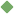vertical_angle 1. [ noun ] (mathematics,geometry) either of two equal and opposite angles formed by the intersection of two straight lines Related terms: angle To share this definition press "text" (Facebook, Twitter) or "link" (blog, mail) then paste text link Similar spelling: vertical_file   vertical_fin   vertical_circle   vertical_tail   vertical_bank   vertical_union   vertical_flute   verticalness   vertical_surface   vertical_section   verticality   verticillate   verticillated